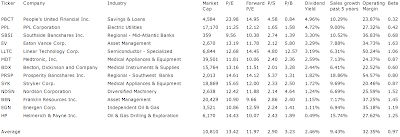## Pages

### Best Dividend Achiever Picks For 2012

Top Dividend Achiever To Buy For 2012 by Dividend Yield - Stock, Capital, Investment. Here is a current sheet of Dividend Achiever (stocks that have raised dividends for at least 10 year in a row without a break) with an interesting market valuation, a great growth performance as well as good earnings situation. Such stocks have a forward price to earnings ratio of less than 15, a sales growth over the past five years of more than 5 percent as well as an operating margin above 20 percent. Exactly 13 stocks with a market capitalization of more than USD 1 billion fulfilled these criteria. Five of them have a yield above 3 percent.

Here are the 3 top dividend stocks by dividend yield:

People's United Financial (NASDAQ:PBCT) has a market capitalization of \$4.58 billion. The company employs 4,528 people, generates revenues of \$828.80 million and has a net income of \$85.70 million. The firm’s earnings before interest, taxes, depreciation and amortization (EBITDA) amounts to \$144.00 million. Because of these figures, the EBITDA margin is 17.39 percent (operating margin 12.72 percent and the net profit margin finally 8.59 percent).

The total debt representing 2.73 percent of the company’s assets and the total debt in relation to the equity amounts to 13.10 percent. Due to the financial situation, the return on equity amounts to 1.63 percent. Twelve trailing months earnings per share amounts to \$0.53. Last fiscal year, the company paid \$0.62 in form of dividends to shareholders.

Here are the price ratios of the company: The P/E ratio is 23.81, Price/Sales 4.64 and Price/Book ratio 0.88. Dividend Yield: 4.91 percent. The beta ratio is 0.32.Long-Term Stock Chart Of People's United Financ... (Click to enlarge)

PPL Corporation (NYSE:PPL) has a market capitalization of \$17.17 billion. The company employs 13,809 people, generates revenues of \$8,521.00 million and has a net income of \$976.00 million. The firm’s earnings before interest, taxes, depreciation and amortization (EBITDA) amounts to \$2,419.00 million. Because of these figures, the EBITDA margin is 28.39 percent (operating margin 21.86 percent and the net profit margin finally 11.45 percent).

The total debt representing 40.68 percent of the company’s assets and the total debt in relation to the equity amounts to 162.69 percent. Due to the financial situation, the return on equity amounts to 13.88 percent. Twelve trailing months earnings per share amounts to \$2.64. Last fiscal year, the company paid \$1.40 in form of dividends to shareholders.

Here are the price ratios of the company: The P/E ratio is 11.23, Price/Sales 2.02 and Price/Book ratio 1.75. Dividend Yield: 4.70 percent. The beta ratio is 0.42.Long-Term Stock Chart Of PPL Corporation (Click to enlarge)

Southside Bancshares (NASDAQ:SBSI) has a market capitalization of \$358.79 million. The company employs 578 people, generates revenues of \$131.37 million and has a net income of \$40.45 million. The firm’s earnings before interest, taxes, depreciation and amortization (EBITDA) amounts to \$78.75 million. Because of these figures, the EBITDA margin is 59.95 percent (operating margin 38.13 percent and the net profit margin finally 29.42 percent).

The total debt representing 2.23 percent of the company’s assets and the total debt in relation to the equity amounts to 31.17 percent. Due to the financial situation, the return on equity amounts to 18.98 percent. Twelve trailing months earnings per share amounts to \$2.28. Last fiscal year, the company paid \$0.81 in form of dividends to shareholders.

Here are the price ratios of the company: The P/E ratio is 9.57, Price/Sales 2.64 and Price/Book ratio 1.69. Dividend Yield: 3.26 percent. The beta ratio is 0.68.Long-Term Stock Chart Of Southside Bancshares, ... (Click to enlarge)

Here is the full table with some fundamentals (TTM):Best Dividend Achiever Picks For 2012 (Click to enlarge)

Take a closer look at the full table. The average price to earnings ratio (P/E ratio) amounts to 13.42 while the forward price to earnings ratio is 11.97. The dividend yield has a value of 2.46 percent. Price to book ratio is 3.23 and price to sales ratio 2.90. The operating margin amounts to 32.35 percent.

Related stock ticker symbols:
PBCT, PPL, SBSI, EV, LLTC, MDT, BDX, PRSP, SYK, NDSN, BEN, EGN, HP

Selected Articles: